Ratio and Proportion. First two years of secondary education.

Direct proportion.

1. The other day I went to the local fruit shop with my dad to buy two kilos of oranges. The two kilos cost 1 and there were twelve oranges altogether. My mother has asked me to go to the fruit shop again today to buy some more oranges as there aren't any left. I want to buy 5 kilos this time. How much will it cost me?

Use the following window to solve the problem.

The x-axis (horizontal) represents the kilos of oranges and the y-axis (vertical) the price. Change the kilo variable to 5 and look at the price given. Work out the price of the following quantities of oranges in the same way: 1, 2, 3, 4, 6, 7 and 10 kilos.

Draw a table like the one below into your exercise book and write in the results.

 Kilos of oranges 1 2 3 4 5 6 7 10 Price in Euros

You should have noticed a relationship between the weight of the oranges and their price: two kilos of oranges cost 1. Double the amount of oranges costs 2, which is double the price; triple the amount costs 3, which is triple the price; one kilo costs half a euro (0.50) etc. We could say that these two quantities (the weight and price) are proportional. Note that the ratio between the price of the oranges and their weight is always the same, or constant at 0.5.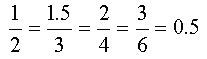When two fractions are equal to each other we can say that they are in proportion.

The quotient of the fractions is called the proportionality constant.

2. How many oranges are there altogether? Is the number of oranges proportional to their weight?

Complete the following table and check the results in the window.

Write the proportionality constant into your exercise book.

 Kilos of oranges 1 2 3 4 5 6 7 10 Nº of oranges

The graph of the function which relates two quantities which are in proportion is always a straight line which goes through the origin.

There are several quantities or magnitudes in everyday life which are proportional and many that are not. Look at the relations between different magnitudes listed below and decide which are proportional and which are not.

1. The price and weight of a sack of potatoes.
2. The price and number of pages of a book.
3. The number of pages in a book and the time taken to read them.
4. The volume and weight of water.
5. The length of the circumference and radius of a circle.
6. The perimeter of a square and the length of its sides.
7. The are of a square and the length of its sides.
8. The weight and age of a baby.

 The basic relationship between two proportions. In the ratio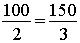the numbers 100 and 3 are called the extreme terms of the proportion, whereas the number 150 and 2 are called the mean terms. Note that the product of the mean terms (150 · 2 = 300) is equal to the product of the extreme terms (100 · 3 = 300).  This relation is true in any ratio; i.e.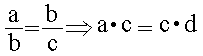In a proportional relationship the product of the mean terms is equal to the product of the extreme terms. Check that the above relationship is satisfied by using the results given for the proportions in the last two exercises.

The rule of  3 : direct proportion.

3. A car takes 4 hours to travel 360 km. If it continues to travel at this speed how far will it have travelled in 5 hours?

If the speed of the car is constant it is easy to see that the distance travelled and the time taken to do so are proportional. Because of this, the fractions obtained by dividing the distance travelled by the time taken (or vice versa: time divided by distance) are equivalent fractions and we can apply the relationship given above to these proportions. Therefore:

360 / 4 = x / 5

where x is the distance travelled in 5 hours.

Look at the results obtained in the following window.

 Change the values of the variables to help you solve this next problem:

4. If the AVE (a high-speed train) takes 2 hours to travel a distance of 400 km from Madrid to Cordoba, how far will it have travelled in 3 hours?

You should apply exactly the same method when you want to solve similar kinds of problems. It is easy to work out the fourth value when you know 3 of the 4 values in the relationship above.

Try out the method to solve the following problems:

Check your answers in the following window by assigning the variables a, b and c their corresponding values.

5. If a cyclist cycles 20 km in 40 minutes how far would they cycle in an hour (60 minutes)?

6. If a bottle of lemonade costs 0.45 how much would a box of 12 bottles cost?

7. If there are 24 hours in a day how many hours are there in a week?

8. If a packet with 5 sticks of chewing gum costs 0.75 how much do 3 packets cost? How many packets could you buy for 3?

9. If a euro is worth 166.386 pesetas how many pesetas are equivalent to 5 euros? How many euros are equal to 1,000 pesetas?

Inverse proportion.

There are relationships where as one quantity decreases the other increases. One example of this is when we travel by car. The faster the speed, the less time it takes us to travel a certain distance.

This particular relationship between these two quantities is also a special case. If the speed is doubled the time taken is halved, if the speed is tripled the time taken is a third, etc.

When this type of relationship is satisfied we can say that the two quantities are inversely proportional.

10. A school wants to organise a school trip in the Spring. The school hires an 80-seater bus and chauffeur for 360. If the school fills the bus how much should each student pay? How much would each student have to pay if the bus was only half full?

Assign the corresponding values for the amount of seats filled on the bus in the following window and look carefully at the results given. Complete the following table with the results.

 Seats filled 10 20 30 40 50 60 70 80 Price per student

 The rule of 3 : inverse proportion. It is easy to work out that if all the seats are filled each student would have to pay 360/80 = 4.50. If only 40 seats are filled we do the following:     If for 80 seats each student pays 4.50         for 40 seats each student pays  x. Now, as the relationship involves inverse proportion we have to invert the second fraction. Therefore: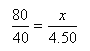After carrying out the necessary operations we find that x= (4.50 * 80) / 40 =  9. Use the rule of 3 method to work out whether the values in the table above are correct or not.Use it to solve the following problems too: 11. Half way through calling the football pools results the radio announces that so far 6 people have predicted all the score draws correctly and that each winner will receive 108,000. By the time they finish calling the results however, they announce that 9 people guessed the exact results instead. How much will each winner now win? 12. A bricklayer takes 5 days to build a wall of 84 m². How long would it take 3 bricklayers to build the same wall working at the same rate?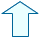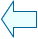Fernando Arias Fernández-PérezSpanish Ministry of Education. Year 2001